# scipy.spatial.distance.yule¶

scipy.spatial.distance.yule(u, v)[source]

Computes the Yule dissimilarity between two boolean 1-D arrays.

The Yule dissimilarity is defined as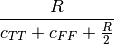where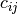is the number of occurrences of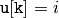and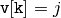for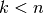and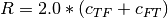.

Parameters : u : (N,) array_like, bool Input array. v : (N,) array_like, bool Input array. yule : double The Yule dissimilarity between vectors u and v.

#### Previous topic

scipy.spatial.distance.wminkowski

#### Next topic

scipy.spatial.KDTree